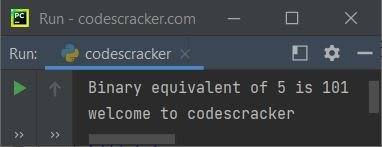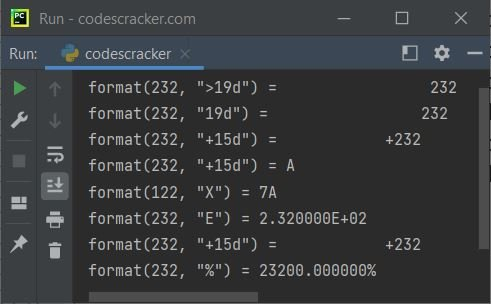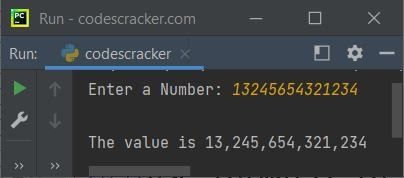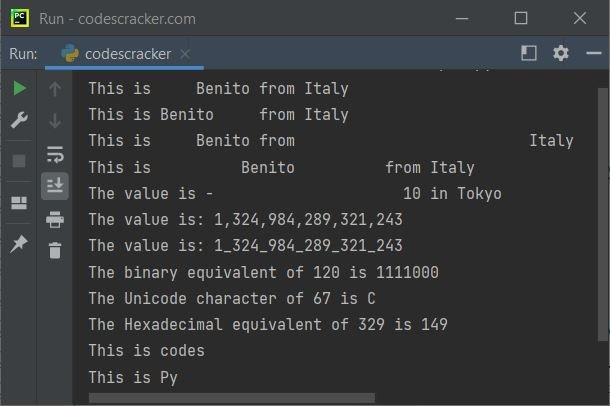# Python format() Function

This article is created to cover format() function of Python in both ways. That is, one to use format() for number formatting, whereas second to use format() for string formatting.

The format() function in Python is used when we need to format the specified value. For example:

```x = 5
xBin = format(x, 'b')
print("Binary equivalent of", x, "is", xBin)

a = "welcome"
b = "codes"
c = "cracker"
x = "{} to {}{}".format(a, b, c)
print(x)```

The snapshot given below shows the sample output produced by this Python program, demonstrating the format() function:In above program, the first format() function is used to format the specified number. Whereas the second format() is used to format the string. The program can also be created in this way:

```x = 5
xBin = format(x, 'b')
print("Binary equivalent of", x, "is", xBin)

sn = "codescracker"
print("welcome to {}".format(sn))```

## Python format() Function Syntax - Number

Since we're talking about both type of format() function, that is one to format a number, and other to format a string. The syntax of format() that returns the formatted version of the specified value using the format specifier, is:

`format(val[, fs])`

where val refers to the value that has to be formatted, whereas fs refers to the format specifier that defines in/to what way, the value should be formatted.

The second argument of format(), the format specifier must be in the following form:

`[[fill]align][sign][#][width][,][.precision][type]`

where:

• fill - a character
• align
• < - align to the right. Right padding
• > - align to the left. Left padding
• = - to place the plus/minus sign at the left most position
• ^ - align to the center of remaining space. Center padding
• sign
• + - to add the plus sign at left
• - - to add minus sign at left
• width - integer value to define the width
• precision - integer value to define the precision
• type
• b - to format the value in binary format
• c - to format the value in equivalent Unicode character
• d - to format the value in decimal format
• n - similar to 'd' except it uses current locale setting for number separator
• o - to format the value in octal format
• x - to format the value in hexadecimal format (lowercase)
• X - to format the value in hexadecimal format (uppercase)
• e - to format the value in exponential notation (lowercase)
• E - to format the value in exponential notation (uppercase)
• f - to format the value to display fixed point number. The default is 6
• F - similar to 'f' except it displays uppercase 'INF' and 'NAN' instead of 'inf', and 'nan'
• g - to round value to p significant digits. The default precision is 6
• G - similar to 'g' except it uses uppercase 'E', in case if value (number) is large
• % - used to multiply by 100 and adds % at the end

## Python format() Function Example - Number

Here is a simple example uses format() function to format the number. This program uses multiple ways to format the number:

```print("format(232, \">19d\") =", format(232, ">19d"))
print("format(232, \"19d\") =", format(232, "19d"))
print("format(232, \"+15d\") =", format(232, "+15d"))
print("format(232, \"+15d\") =", format(65, "c"))
print("format(122, \"X\") =", format(122, "X"))
print("format(232, \"E\") =", format(232, "E"))
print("format(232, \"+15d\") =", format(232, "+15d"))
print("format(232, \"%\") =", format(232, "%"))```

The sample output of this program is shown in the snapshot given below:### Use format() to Separate every Thousandth Digit by Comma

This program is created to demonstrates how the format() function can be used to format a number in a way to separate every thousandth digit by comma.

`print(format(13232433432, ",d"))`

The output will be:

`13,232,433,432`

The above program can also be created in this way. This program allows user to define the number:

```print("Enter a Number: ", end="")
num = int(input())
print("\nThe value is {:,}".format(num))```

The sample run with user input 13245654321234 is shown in the snapshot given below:Note: The above program uses String.format() function. The detailed description of formatting string using format() is given below.

## Python format() Function Syntax - String

The syntax of format() function to format the string in Python, is:

`str.format(val1, val2, val3, ..., valN)`

where str refers to the string. And val1, val2, and so on refers to values. Parameter(s) to format() function to format the string, is required. Parameter may be comprises of a single or multiple values. The values are:

• either some list of values, separate by commas
• or some list of values in the form of key=value pairs, separated by commas

Note: Parameter's value of format() function can be of any data type.

The string must contain {} (acts as placeholder for the value) for equal number parameters. For example, if there are 5 parameters, then string must contains five {} to put all parameters inside this curly braces (acts as placeholder for format() parameter's value) in the string.

## Python format() Function Example - String

This is a simple example program, demonstrates the format() function:

```x = "Python"
print("Welcome to {} Programming.".format(x))```

The output will be:

`Welcome to Python Programming.`

### Use format() without Indexing in Python

This program uses normal way of the format() function to format the string, without indexing:

```a = "Alicia"
b = "EECS"
c = "University of California"
d = "Berkeley"

print("This is {}, studying {} at {}, {}".format(a, b, c, d))```

The output will be:

`This is Alicia, studying EECS at University of California, Berkeley`

### Use format() with Indexing in Python

This program shows how the format() function can be used with indexing:

```a = "Alicia"
b = "EECS"
c = "University of California"
d = "Berkeley"

print("This is {3}, studying {2} at {1}, {0}".format(d, c, b, a))```

The output would be same as of previous program's output. In above program:

• the first parameter of format() function is d whose index is 0
• the second parameter is c whose index is 1
• the third parameter is b whose index is 2
• and the fourth parameter is a whose index is 3

Therefore putting 3 inside {} will consider the value at index 3 (means the value of a will get placed to {3} in above program). Same things goes with others.

### Parameter(s) of format() in Key=Value Pair(s)

This program is created in a way to use format() function with its parameter in the form of key=value pair(s).

`print("This is {a}, studying {b}".format(a="Alicia", b="EECS"))`

The output will be:

`This is Alicia, studying EECS`

Here is another example program:

```c = "University of California"
d = "Berkeley"
print("This is {a}, studying {b} at {c}, {d}".format(a="Alicia", b="EECS", c=c, d=d))```

The output will be:

`This is Alicia, studying EECS at University of California, Berkeley`

### Python String.format() Example

This is the last example program of format() function to format the string in different-different way:

```print("This is {:>10} from Italy".format("Benito"))
print("This is {:<10} from Italy".format("Benito"))
print("This is {:>10} from {:>30}".format("Benito", "Italy"))
print("This is {:^24} from Italy".format("Benito"))
print("The value is {:=24} in Tokyo".format(-10))
print("The value is: {:,}".format(1324984289321243))
print("The value is: {:_}".format(1324984289321243))
print("The binary equivalent of 120 is {:b}".format(120))
print("The Unicode character of 67 is {:c}".format(67))
print("The Hexadecimal equivalent of 329 is {:X}".format(329))
print("This is {:.5}".format("codescracker"))
print("This is {:.2}".format("Python Programming"))```

The sample output is shown in the snapshot given below:Note: The {:.5} is used when we need to print only first five character of the string specified as parameter of format() function, for this placeholder.

Python Online Test

« Previous Function Next Function »